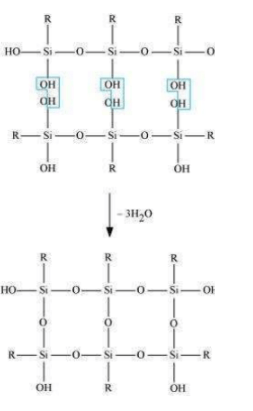Most Affordable JEE | NEET | 8,9,10 Preparation by Kota's Top IITian Doctor Faculties

# NCERT Solutions for Class 11 Chemistry chapter 11 The p-block Elements PDF - eSaral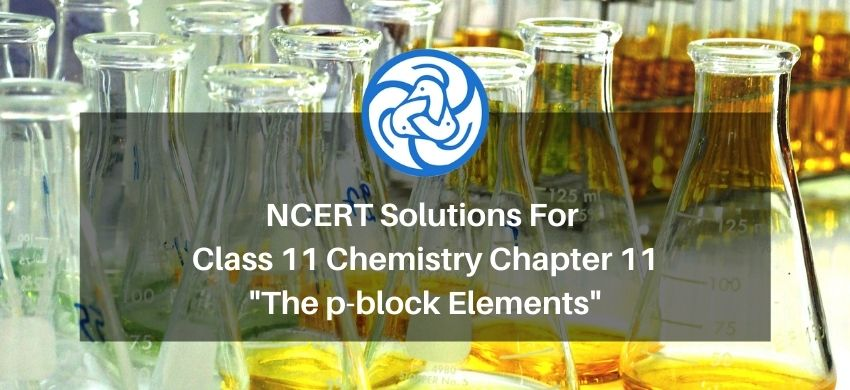`

# NCERT Solutions for Class 11 Chemistry chapter 11 The p-block Elements PDF

Hey, are you a class 11 student and looking for ways to download NCERT Solutions for Class 11 Chemistry chapter 11 The p-block Elements PDF? If yes. Then read this post till the end.

In this article, we have listed NCERT Solutions for Class 11 Chemistry chapter 11 The p-block Elements in PDF that are prepared by Kota’s top IITian’s Faculties by keeping Simplicity in mind.

If you want to learn and understand class 11 Chemistry chapter 11 "The p-block Elements" in an easy way then you can use these solutions PDF.

NCERT Solutions helps students to Practice important concepts of subjects easily. Class 11 Chemistry solutions provide detailed explanations of all the NCERT questions that students can use to clear their doubts instantly.

If you want to score high in your class 11 Chemistry Exam then it is very important for you to have a good knowledge of all the important topics, so to learn and practice those topics you can use eSaral NCERT Solutions.

So, without wasting more time Let’s start.

### Download NCERT Solutions for Class 11 Chemistry chapter 11 The p-block Elements PDF

Question 1: Discuss the pattern of variation in the oxidation states of

(i) $\mathrm{B}$ to $\mathrm{TI}$

And

(ii) $C$ to $P b$.

Solution. (i) $\mathbf{B}$ to $\mathbf{T I}$

The electric configuration of group 13 elements is $n s^{2} n p^{1}$. Therefore, the most common oxidation state exhibited by them should be $+3$. However, it is only boron and aluminium which practically show the $+3$ oxidation state. The remaining elements, i.e., Ga, In, $T$, show both the $+1$ and $+3$ oxidation states. On moving down the group, the $+1$ state becomes more stable. For example, $T 1(+1)$ is more stable than $\mathrm{Tl}(+3)$. This is because of the inert pair effect. The two electrons present in the s-shell are strongly attracted by the nucleus and do not participate in bonding. This inert pair effect becomes more and more prominent on moving down the group. Hence, Ga $(+1)$ is unstable, In $(+1)$ is fairly stable, and $\mathrm{TI}(+1)$ is very stable.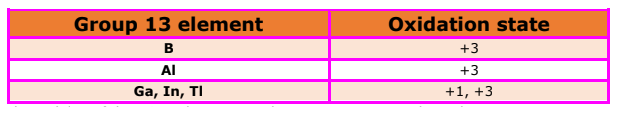The stability of the $+3$ oxidation state decreases on moving down the group.

(ii) $\mathbf{C}$ to $\mathbf{P b}$

The electronic configuration of group 14 elements is $n s^{2} n p^{2}$. Therefore, the most common oxidation state exhibited by them should be $+4$. However, the $+2$ oxidation state becomes more and more common on moving down the group. $\mathrm{C}$ and $\mathrm{Si}$ mostly show the $+4$ state. On moving down the group, the higher oxidation state becomes less stable. This is because of the inert pair effect. Thus, although $\mathrm{Ge}, \mathrm{Sn}$, and $\mathrm{Pb}$ show both the $+2$ and $+4$ states, the stability of the lower oxidation state increases and that of the higher oxidation state decreases on moving down the group.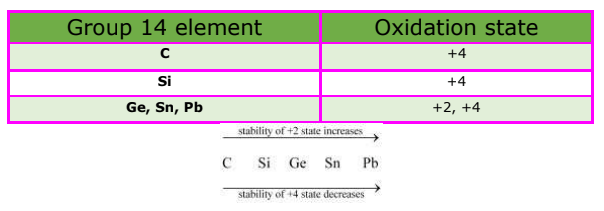Question 2: How can you explain higher stability of $\mathrm{BCl}_{3}$ as compared to $\mathrm{TICl}_{3}$ ?

Solution. Boron and thallium belong to group 13 of the periodic table. In this group, the $+1$ oxidation state becomes more stable on moving down the group. $\mathrm{BCl}_{3}$ is more stable than $\mathrm{TICl}_{3}$ because the $+3$ oxidation state of $\mathrm{B}$ is more stable than the $+3$

oxidation state of $\mathrm{TI}$. In $\mathrm{Tl}$, the $+3$ state is highly oxidising and it reverts back to the more stable $+1$ state.

Question 3: Why does boron trifluoride behave as a Lewis acid?

Solution. The electric configuration of boron is $n s^{2} n p^{1} .$ It has three electrons in its valence shell. Thus, it can form only three covalent bonds. This means that there are only six electrons around boron and its octet remains incomplete. When one atom of boron combines with three fluorine atoms, its octet remains incomplete. Hence, boron trifluoride remains electron-deficient and acts as a Lewis acid.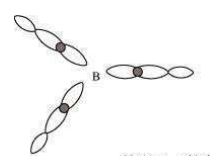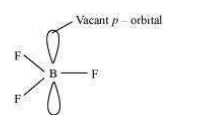Question 4: Consider the compounds, $\mathrm{BCl}_{3}$ and $\mathrm{CCl}_{4}$. How will they behave with water? Justify.

Solution. Being a Lewis acid, $\mathrm{BCl}_{3}$ readily undergoes hydrolysis. Boric acid is formed as a result.

$\mathrm{BCl}_{3}+3 \mathrm{H}_{2} \mathrm{O} \longrightarrow 3 \mathrm{HCl}+\mathrm{B}(\mathrm{OH})_{3}$

$\mathrm{CCl}_{4}$ completely resists hydrolysis. Carbon does not have any vacant orbital. Hence, it cannot accept electrons from water to form an intermediate. When $\mathrm{CCl}_{4}$ and water are mixed, they form separate layers.

$\mathrm{CCl}_{4}+\mathrm{H}_{2} \mathrm{O} \longrightarrow$ No reaction

Question 5: Is boric acid a protic acid? Explain.

Solution. Boric acid is not a protic acid. It is a weak monobasic acid, behaving as a Lewis acid.

$\mathrm{B}(\mathrm{OH})_{3}+2 \mathrm{HOH} \longrightarrow\left[\mathrm{B}(\mathrm{OH})_{4}\right]^{-}+\mathrm{H}_{3} \mathrm{O}^{+}$

It behaves as an acid by accepting a pair of electrons from - OH ion.

Question 6: Explain what happens when boric acid is heated.

Solution. On heating orthoboric acid $\left(\mathrm{H}_{3} \mathrm{BO}_{3}\right)$ at $370 \mathrm{~K}$ or above, it changes to metaboric acid $\left(\mathrm{HBO}_{2}\right)$.

On further heating, this yields boric oxide $\mathrm{B}_{2} \mathrm{O}_{3}$.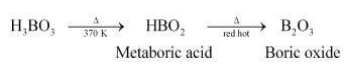Question 7: Describe the shapes of $\mathrm{BF}_{3}$ and $\mathrm{BH}_{4}{ }^{-} .$ Assign the hybridisation of boron in these species.

Solution. (i) $\mathbf{B F}_{3}$

As a result of its small size and high electronegativity, boron tends to form monomeric covalent halides. These halides have a planar triangular geometry. This triangular shape is formed by the overlap of three $s p^{2}$ hybridised orbitals of boron with the sp orbitals of three halogen atoms. Boron is $s p^{2}$ hybridised in $\mathrm{BF}_{3} .$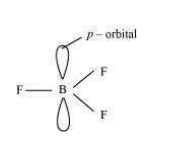(ii) $\mathbf{B H}_{4}^{-}$

Boron-hydride ion $\left(\mathrm{BH}_{4}^{-}\right)$ is formed by the $s p^{3}$ hybridisation of boron orbitals. Therefore, it is tetrahedral in structure.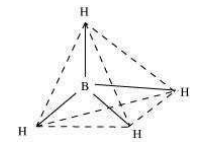Question 8: Write reactions to justify amphoteric nature of aluminium.

Solution. A substance is called amphoteric if it displays characteristics of both acids and bases.

Aluminium dissolves in both acids and bases, showing amphoteric behaviour.

(i) $2 \mathrm{Al}_{(x)}+6 \mathrm{HCl}_{(\infty)} \longrightarrow 2 \mathrm{Al}^{3+}{(\infty)}+6 \mathrm{Cl}_{(\alpha)}^{-}+3 \mathrm{H}_{2(g)}$

(ii) $2 \mathrm{Al}_{(s)}+2 \mathrm{NaOH}_{(\infty)}+6 \mathrm{H}_{2} \mathrm{O}_{(k)}$

$\longrightarrow 2 \mathrm{Na}^{+}\left[\mathrm{Al}(\mathrm{OH})_{4}\right]_{(\infty)}^{-}+3 \mathrm{H}_{2(g)}$

Question 9: What are electron deficient compounds? Are $\mathrm{BCl}_{3}$ and $\mathrm{SiCl}_{4}$ electron deficient species?

Explain.

Solution. In an electron-deficient compound, the octet of electrons is not complete, i.e., the central metal atom has an incomplete octet. Therefore, it needs electrons to complete its octet.

(i) $\mathrm{BCl}_{3}$

$\mathrm{BCl}_{3}$ is an appropriate example of an electron-deficient compound. $\mathrm{B}$ has 3 valence electrons. After forming three covalent bonds with chlorine, the number of electrons around it increases to $6 .$ However, it is still short of two electrons to complete its octet.

(ii) $\mathrm{SiCl}_{4}$

The electronic configuration of silicon is $n s^{2} n p^{2}$. This indicates that it has four valence electrons. After it forms four covalent bonds with four chlorine atoms, its electron count increases to eight. Thus, $\mathrm{SiCl}_{4}$ is not an electron-deficient compound.

Question 10: Write the resonance structures of $\mathrm{CO}_{3}^{2-}$ and $\mathrm{HCO}_{3}^{-} .$

Solution. (a) $\mathrm{CO}_{3}^{2-}$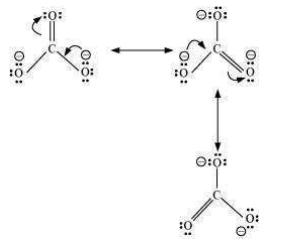(b) $\mathrm{HCO}_{3}^{-}$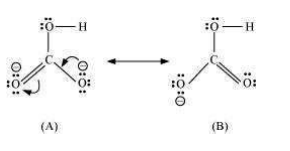There are only two resonating structures for the bicarbonate ion.

Question 11: What is the state of hybridisation of carbon in (a) $\mathrm{CO}_{3}^{2-}$ (b) diamond (c) graphite?

Solution. The state of hybridisation of carbon in:

(a) $\mathrm{CO}_{3}^{2-}$

$\mathrm{C}$ in $\mathrm{CO}_{3}^{2-}$ is $s p^{2}$ hybridised and is bonded to three oxygen atoms.

(b) Diamond

Each carbon in diamond is $s p^{3}$ hybridised and is bound to four other carbon atoms.

(c) Graphite

Each carbon atom in graphite is $s p^{2}$ hybridised and is bound to three other carbon atoms.

Question 12: Explain the difference in properties of diamond and graphite on the basis of their structures.

Solution.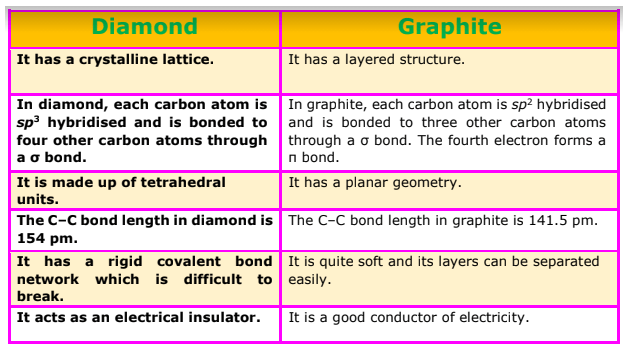Question 13: Rationalise the given statements and give chemical reactions:

- Lead (II) chloride reacts with $\mathrm{Cl}_{2}$ to give $\mathrm{PbCl}_{4}$.

- Lead (IV) chloride is highly unstable towards heat.

- Lead is known not to form an iodide, $\mathrm{PbI}_{4}$.

Solution. (a) Lead belongs to group 14 of the periodic table. The two oxidation states displayed by this group is $+2$ and $+4$. On moving down the group, the $+2$ oxidation state becomes more stable and the $+4$ oxidation state becomes less stable. This is because of the inert pair effect. Hence, $\mathrm{PbCl}_{4}$ is much less stable than $\mathrm{PbCl}_{2}$. However, the formation of $\mathrm{PbCl}_{4}$ takes place when chlorine gas is bubbled through a saturated solution of $\mathrm{PICl}_{2}$.

$\mathrm{PbCl}_{2(n)}+\mathrm{Cl}_{2(g)} \longrightarrow \mathrm{PbCl}_{4()}$

(b) On moving down group IV, the higher oxidation state becomes unstable because of the inert pair effect. Pb(IV) is highly unstable and when heated, it reduces to $\mathrm{Pb}(\mathrm{II})$.

$\mathrm{PbCl}_{4(1)} \stackrel{\triangle}{\longrightarrow} \mathrm{PbCl}_{2(3)}+\mathrm{Cl}_{2(g)}$

(c) Lead is known not to form PbI4. $P b(+4)$ is oxidising in nature and $\mathrm{I}^{-}$ is reducing in nature. A combination of Pb(IV) and iodide ion is not stable. Iodide ion is strongly reducing in nature. $\mathrm{Pb}(\mathrm{IV})$ oxidises $\mathrm{I}^{-}$ to $\mathrm{I}^{2}$ and itself gets reduced to $\mathrm{Pb}(\mathrm{II})$.

$\mathrm{PbI}_{4} \longrightarrow \mathrm{Pbl}_{2}+\mathrm{I}_{2}$

Question 14: Suggest reasons why the B-F bond lengths in BF $(130 \mathrm{pm})$ and $B F_{4}^{-}$ ( $143 \mathrm{pm}$ ) differ.

Solution. The B-F bond length in $\mathrm{BF}_{3}$ is shorter than the $\mathrm{B}-\mathrm{F}$ bond length in $B F_{4}^{-} . \mathrm{BF}_{3}$ is an electrondeficient species. With a vacant $p$ -orbital on boron, the fluorine and boron atoms undergo pп-pп back-bonding to remove this deficiency. This imparts a double bond character to the B-F bond.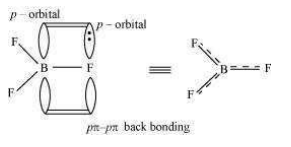This double-bond character causes the bond length to shorten in $\mathrm{BF}_{3}(130 \mathrm{pm}) .$ However, when BFa coordinates with the fluoride ion, a change in hybridisation from $s p^{2}$ (in BF 3 ) to $s p^{3}$ (in $B F_{4}^{-}$ ) occurs. Boron now forms $4 \sigma$ bonds and the double-bond character is lost. This accounts for a B-F bond length of $143 \mathrm{pm}$ in $B F_{4}^{-}$ ion.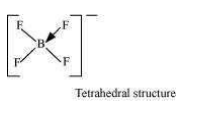Question 15: If $\mathrm{B}-\mathrm{Cl}$ bond has a dipole moment, explain why $\mathrm{BCl}_{3}$ molecule has zero dipole moment.

Solution. As a result of the difference in the electronegativities of $\mathrm{B}$ and $\mathrm{Cl}$, the $\mathrm{B}-\mathrm{Cl}$ bond is polar in nature. However, the $\mathrm{BCl}_{3}$ molecule is non-polar. This is because $\mathrm{BCl}_{3}$ is trigonal planar in shape. It is a symmetrical molecule. Hence, the respective dipole-moments of the $\mathrm{B}-\mathrm{Cl}$ bond cancel each other, thereby causing a zero-dipole moment.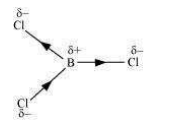Question 16: Aluminium trifluoride is insoluble in anhydrous HF but dissolves on addition of NaF. Aluminium trifluoride precipitates out of the resulting solution when gaseous $\mathrm{BF}_{3}$ is bubbled through. Give reasons.

Solution. Hydrogen fluoride (HF) is a covalent compound and has a very strong intermolecular

hydrogen-bonding. Thus, it does not provide ions and aluminium fluoride (AIF) does not dissolve in it. Sodium fluoride (NaF) is an ionic compound and when it is added to the mixture, AIF dissolves. This is because of the availability of free $F^{-}$. The reaction involved in the process is: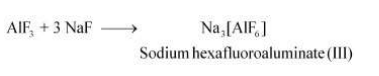When boron trifluoride $\left(\mathrm{BF}_{3}\right)$ is added to the solution, aluminium fluoride precipitates out of the solution. This happens because the tendency of boron to form complexes is much more than that of aluminium. Therefore, when $\mathrm{BF}_{3}$ is added to the solution, $\mathrm{B}$ replaces $\mathrm{Al}$ from the complexes according to the following reaction:

$\mathrm{Na}_{3}\left[\mathrm{AlF}_{6}\right]+3 \mathrm{BF}_{3} \longrightarrow 3 \mathrm{Na}\left[\mathrm{BF}_{4}\right]+\mathrm{AlF}_{3}$

Question 17: Suggest a reason as to why CO is poisonous.

Solution. Carbon monoxide is highly-poisonous because of its ability to form a complex with haemoglobin. The CO-Hb complex is more stable than the $\mathrm{O}_{2}-\mathrm{Hb}$ complex. The former prevents $\mathrm{Hb}$ from binding with oxygen. Thus, a person dies because of suffocation on not receiving oxygen. It is found that the

CO-Hb complex is about 300 times more stable than the $\mathrm{O}_{2}-\mathrm{Hb}$ complex.

Question 18: How is excessive content of $\mathrm{CO}_{2}$ responsible for global warming?

Solution. Carbon dioxide is a very essential gas for our survival. However, an increased content of $\mathrm{CO}_{2}$ in the atmosphere poses a serious threat. An increment in the combustion of fossil

fuels, decomposition of limestone, and a decrease in the number of trees has led to greater levels of carbon dioxide. Carbon dioxide has the property of trapping the heat provided by sunrays. Higher the level of carbon dioxide, higher is the amount of heat trapped. This results in an increase in the atmospheric temperature, thereby causing global warming.

Question 19: Explain structures of diborane and boric acid.

Solution. (a) Diborane

$\mathrm{B}_{2} \mathrm{H}_{6}$ is an electron-deficient compound. $\mathrm{B}_{2} \mathrm{H}_{6}$ has only 12 electrons $-6 \mathrm{e}^{-}$ from $6 \mathrm{H}$ atoms and $3 \mathrm{e}^{-}$ each from $2 \mathrm{~B}$ atoms. Thus, after combining with $3 \mathrm{H}$ atoms, none of the boron atoms has any electrons left. X-ray diffraction studies have shown the structure of

diborane as: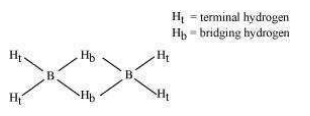2 boron and 4 terminal hydrogen atoms $(\mathrm{Ht})$ lie in one plane, while the other two bridging hydrogen atoms $\left(\mathrm{H}_{\mathrm{b}}\right)$ lie in a plane perpendicular to the plane of boron atoms. Again, of the two bridging hydrogen atoms, one $\mathrm{H}$ atom lies above the plane and the other lies below the plane. The terminal bonds are regular two-centre two-electron $\left(2 c-2 e^{-}\right)$ bonds, while the two bridging $(\mathrm{B}-\mathrm{H}-\mathrm{B})$ bonds are three-centre two-electron $\left(3 \mathrm{c}-2 \mathrm{e}^{-}\right)$ bonds.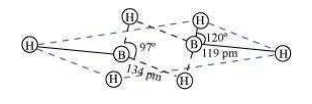(b) Boric acid

Boric acid has a layered structure. Each planar $\mathrm{BO}_{3}$ unit is linked to one another through $\mathrm{H}$ atoms. The $\mathrm{H}$ atoms form a covalent bond with a $\mathrm{BO}_{3}$ unit, while a hydrogen bond is formed with another $\mathrm{BO}_{3}$ unit. In the given figure, the dotted lines represent hydrogen bonds.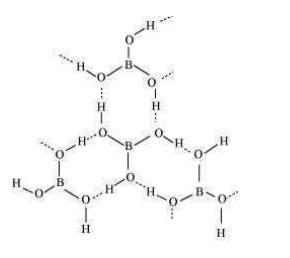Question 20: What happens when

(a) Borax is heated strongly,

(b) Boric acid is added to water,

(c) Aluminium is treated with dilute $\mathrm{NaOH}$,

(d) BF $_{3}$ is reacted with ammonia?

Solution. (a) When heated, borax undergoes various transitions. It first loses water molecules and swells. Then, it turns into a transparent liquid, solidifying to form a glass-like material called borax bead.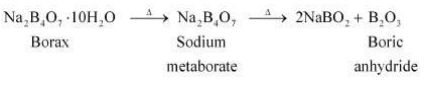(b) When boric acid is added to water, it accepts electrons from - OH ion.

$\mathrm{B}(\mathrm{OH})_{3}+2 \mathrm{HOH} \longrightarrow\left[\mathrm{B}(\mathrm{OH})_{4}\right]^{-}+\mathrm{H}_{3} \mathrm{O}^{+}$

(c) Al reacts with dilute $\mathrm{NaOH}$ to form sodium tetrahydroxoaluminate(III). Hydrogen gas is liberated in the process.

$2 \mathrm{Al}_{(\theta)}+2 \mathrm{NaOH}_{(\omega)}+6 \mathrm{H}_{2} \mathrm{O}_{(i)}$

$\longrightarrow 2 \mathrm{Na}^{+}\left[\mathrm{Al}(\mathrm{OH})_{4}\right]_{\left(\alpha_{09}\right)}+3 \mathrm{H}_{2(g)}$

(d) $\mathrm{BF}_{3}$ (a Lewis acid) reacts with $\mathrm{NH}_{3}$ (a Lewis base) to form an adduct. This results in a

complete octet around $\mathrm{B}$ in $\mathrm{BF}_{3}$.

$\mathrm{F}_{3} \mathrm{~B}+\mathrm{NH}_{3} \longrightarrow \mathrm{F}_{3} \mathrm{~B} \leftarrow: \mathrm{NH}_{3}$

Question 21: Explain the following reactions

(a) Silicon is heated with methyl chloride at high temperature in the presence of copper;

(b) Silicon dioxide is treated with hydrogen fluoride;

(c) CO is heated with $\mathrm{ZnO}$;

(d) Hydrated alumina is treated with aqueous $\mathrm{NaOH}$ solution.

Solution. (a) When silicon reacts with methyl chloride in the presence of copper (catalyst) and at a temperature of about $537 \mathrm{~K}$, a class of organosilicon polymers called methylsubstituted

chlorosilanes $\left(\mathrm{MeSiCl}_{3}, \mathrm{Me}_{2} \mathrm{SiCl}_{2}, \mathrm{Me}_{3} \mathrm{SiCl}\right.$, and $\left.\mathrm{Me}_{4} \mathrm{Si}\right)$ are formed.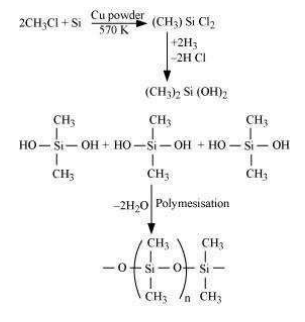(b) When silicon dioxide $\left(\mathrm{SiO}_{2}\right)$ is heated with hydrogen fluoride (HF), it forms silicon tetrafluoride $\left(\mathrm{SiF}_{4}\right) .$ Usually, the Si-O bond is a strong bond and it resists any attack by halogens and most acids, even at a high temperature. However, it is attacked by

HF.

$\mathrm{SiO}_{2}+4 \mathrm{HF} \longrightarrow \mathrm{SiF}_{4}+2 \mathrm{H}_{2} \mathrm{O}$

The $\mathrm{SiF}_{4}$ formed in this reaction can further react with HF to form hydrofluorosilicic acid.

$\mathrm{SiF}_{4}+2 \mathrm{HF} \longrightarrow \mathrm{H}_{2} \mathrm{SiF}_{6}$

(c) When CO reacts with $\mathrm{ZnO}$, it reduces $\mathrm{ZnO}$ to $\mathrm{Zn}$. CO acts as a reducing agent.

$\mathrm{ZnO}_{(s)}+\mathrm{CO}_{(x)} \stackrel{\Delta}{\longrightarrow} \mathrm{Zn}_{(x)}+\mathrm{CO}_{2(g)}$

(d) When hydrated alumina is added to sodium hydroxide, the former dissolves in the latter because of the formation of sodium meta-aluminate.

$\mathrm{Al}_{2} \mathrm{O}_{3} \cdot 2 \mathrm{H}_{2} \mathrm{O}+2 \mathrm{NaOH} \longrightarrow 2 \mathrm{NaAlO}_{2}+3 \mathrm{H}_{2} \mathrm{O}$

Question 22: Give reasons:

(i) Conc. HNOs can be transported in aluminium container.

(ii) A mixture of dilute $\mathrm{NaOH}$ and aluminium pieces is used to open drain.

(iii) Graphite is used as lubricant.

(iv) Diamond is used as an abrasive.

(v) Aluminium alloys are used to make aircraft body.

(vi) Aluminium utensils should not be kept in water overnight.

(vii) Aluminium wire is used to make transmission cables.

Solution. (i) Concentrated $\mathrm{HNO}_{3}$ can be stored and transported in aluminium containers as it reacts with aluminium to form a thin protective oxide layer on the aluminium surface. This oxide layer renders aluminium passive.

(ii) Sodium hydroxide and aluminium react to form sodium tetrahydroxoaluminate(III) and hydrogen gas. The pressure of the produced hydrogen gas is used to open blocked drains.

$2 \mathrm{Al}+2 \mathrm{NaOH}+6 \mathrm{H}_{2} \mathrm{O} \longrightarrow 2 \mathrm{Na}^{+}\left[\mathrm{Al}(\mathrm{OH})_{4}\right]^{-}+3 \mathrm{H}_{2}$

(iii) Graphite has a layered structure and different layers of graphite are bonded to each other by weak van der Waals' forces. These layers can slide over each other. Graphite is soft and slippery. Therefore, graphite can be used as a lubricant.

(iv) In diamond, carbon is $s p^{3}$ hybridised. Each carbon atom is bonded to four other carbon atoms with the help of strong covalent bonds. These covalent bonds are present throughout the surface, giving it a very rigid 3-D structure. It is very difficult to break this extended covalent bonding and for this reason, diamond is the hardest substance known. Thus, it is used as an abrasive and for cutting tools.

(v) Aluminium has a high tensile strength and is very light in weight. It can also be alloyed with various metals such as $\mathrm{Cu}, \mathrm{Mn}, \mathrm{Mg}, \mathrm{Si}$, and $\mathrm{Zn}$. It is very malleable and ductile. Therefore, it is used in making aircraft bodies.

(vi) The oxygen present in water reacts with aluminium to form a thin layer of aluminium oxide. This layer prevents aluminium from further reaction. However, when water is kept in an aluminium vessel for long periods of time, some amount of aluminium oxide may dissolve in water. As aluminium ions are harmful, water should not be stored in aluminium vessels overnight.

(vii) Silver, copper, and aluminium are among the best conductors of electricity. Silver is an expensive metal and silver wires are very expensive. Copper is quite expensive and is also very heavy. Aluminium is a very ductile metal. Thus, aluminium is used in making wires for electrical conduction.

Question 23: Explain why is there a phenomenal decrease in ionisation enthalpy from carbon to silicon?

Solution. Ionisation enthalpy of carbon (the first element of group 14 ) is very high $(1086 \mathrm{~kJ} / \mathrm{mol})$. This is expected owing to its small size. However, on moving down the group to silicon, there is a sharp decrease in the enthalpy $(786 \mathrm{~kJ})$. This is because of an appreciable increase in the atomic sizes of elements on moving down the group.

Question 24: How would you explain the lower atomic radius of Ga as compared to Al?

Solution.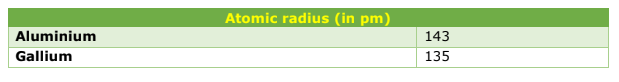Although Ga has one shell more than Al, its size is lesser than Al. This is because of the poor shielding effect of the $3 d$ -electrons. The shielding effect of $d$ -electrons is very poor and the effective nuclear charge experienced by the valence electrons in gallium is much more than it is in the case of Al.

Question 25: What are allotropes? Sketch the structure of two allotropes of carbon namely diamond and graphite. What is the impact of structure on physical properties of two allotropes?

Solution. Allotropy is the existence of an element in more than one form, having the same chemical properties but different physical properties. The various forms of an element are called allotropes.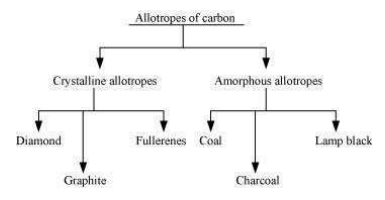Diamond: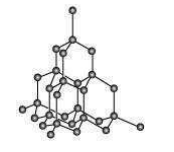The rigid 3-D structure of diamond makes it a very hard substance. In fact, diamond is one of the hardest naturally-occurring substances. It is used as an abrasive and for cutting tools.

Graphite: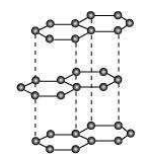It has $s p^{2}$ hybridised carbon, arranged in the form of layers. These layers are held together by weak van der Walls' forces. These layers can slide over each other, making graphite soft and slippery. Therefore, it is used as a lubricant.

Question 26: (a) Classify following oxides as neutral, acidic, basic or amphoteric:

$\mathrm{CO}, \mathrm{B}_{2} \mathrm{O}_{3}, \mathrm{SiO}_{2}, \mathrm{CO}_{2}, \mathrm{Al}_{2} \mathrm{O}_{3}, \mathrm{PbO}_{2}, \mathrm{Tl}_{2} \mathrm{O}_{3}$

(b) Write suitable chemical equations to show their nature.

Solution. (1) $\mathrm{CO}=$ Neutral

(2) $\mathrm{B}_{2} \mathrm{O}_{3}=$ Acidic

Being acidic, it reacts with bases to form salts. It reacts with $\mathrm{NaOH}$ to form sodium metaborate.

$\mathrm{B}_{2} \mathrm{O}_{3}+2 \mathrm{NaOH} \longrightarrow 2 \mathrm{NaBO}_{2}+\mathrm{H}_{2} \mathrm{O}$

(3) $\mathrm{SiO}_{2}=$ Acidic

Being acidic, it reacts with bases to form salts. It reacts with $\mathrm{NaOH}$ to form sodium silicate.

$\mathrm{SiO}_{2}+2 \mathrm{NaOH} \longrightarrow 2 \mathrm{Na}_{2} \mathrm{SiO}_{3}+\mathrm{H}_{2} \mathrm{O}$

(4) $\mathrm{CO}_{2}=$ Acidic

Being acidic, it reacts with bases to form salts. It reacts with $\mathrm{NaOH}$ to form sodium carbonate.

$\mathrm{CO}_{2}+2 \mathrm{NaOH} \longrightarrow \mathrm{Na}_{2} \mathrm{CO}_{3}+\mathrm{H}_{2} \mathrm{O}$

(5) $\mathrm{Al}_{2} \mathrm{O}_{3}=$ Amphoteric

Amphoteric substances react with both acids and bases. $\mathrm{Al}_{2} \mathrm{O}_{3}$ reacts with both $\mathrm{NaOH}$ and $\mathrm{H}_{2} \mathrm{SO}_{4}$.

$\mathrm{Al}_{2} \mathrm{O}_{3}+2 \mathrm{NaOH} \longrightarrow \mathrm{NaAlO}_{2}$

$\mathrm{Al}_{2} \mathrm{O}_{3}+3 \mathrm{H}_{2} \mathrm{SO}_{4} \longrightarrow \mathrm{Al}_{2}\left(\mathrm{SO}_{4}\right)_{3}+3 \mathrm{H}_{2} \mathrm{O}$

(6) $\mathrm{PbO}_{2}=$ Amphoteric

Amphoteric substances react with both acids and bases. $\mathrm{PbO}_{2}$ reacts with both $\mathrm{NaOH}$ and $\mathrm{H}_{2} \mathrm{SO}_{4} .$

$\mathrm{PbO}_{2}+2 \mathrm{NaOH} \longrightarrow \mathrm{Na}_{2} \mathrm{PbO}_{3}+\mathrm{H}_{2} \mathrm{O}$

$2 \mathrm{PbO}_{2}+2 \mathrm{H}_{2} \mathrm{SO}_{4} \longrightarrow 2 \mathrm{PbSO}_{4}+2 \mathrm{H}_{2} \mathrm{O}+\mathrm{O}_{2}$

(7) $\mathrm{Tl}_{2} \mathrm{O}_{3}=$ Basic

Being basic, it reacts with acids to form salts. It reacts with $\mathrm{HCl}$ to form thallium chloride.

$\mathrm{Tl}_{2} \mathrm{O}_{3}+6 \mathrm{HCl} \longrightarrow 2 \mathrm{TICl}_{3}+3 \mathrm{H}_{2} \mathrm{O}$

Question 27: In some of the reactions thallium resembles aluminium, whereas in others it resembles with group I metals. Support this statement by giving some evidences.

Solution. Thallium belongs to group 13 of the periodic table. The most common oxidation state for this group is $+3$. However, heavier members of this group also display the $+1$ oxidation state. This happens because of the inert pair effect. Aluminium displays the $+3$ oxidation state and alkali metals display the $+1$ oxidation state. Thallium displays both the oxidation states. Therefore, it resembles both aluminium and alkali metals.

Thallium, like aluminium, forms compounds such as $\mathrm{TICl}_{3}$ and $\mathrm{T} \mathrm{I}_{2} \mathrm{O}_{3}$. It resembles alkali metals in compounds $\mathrm{Tl}_{2} \mathrm{O}$ and $\mathrm{TICl}$.

Question 28: When metal $X$ is treated with sodium hydroxide, a white precipitate (A) is obtained, which is soluble in excess of $\mathrm{NaOH}$ to give soluble complex (B). Compound (A) is soluble in dilute $\mathrm{HCl}$ to form compound (C). The compound (A) when heated strongly gives (D), which is used to extract metal. Identify $(\mathrm{X}),(\mathrm{A}),(\mathrm{B}),(\mathrm{C})$ and $(\mathrm{D}) .$ Write suitable equations to support their identities.

Solution. The given metal $X$ gives a white precipitate with sodium hydroxide and the precipitate

dissolves in excess of sodium hydroxide. Hence, $X$ must be aluminium.

The white precipitate (compound A) obtained is aluminium hydroxide. The compound $\mathrm{B}$ formed when an excess of the base is added is sodium tetrahydroxoaluminate(III).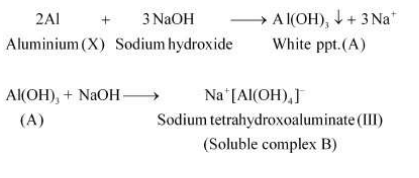Now, when dilute hydrochloric acid is added to aluminium hydroxide, aluminium chloride (compound $\mathrm{C}$ ) is obtained.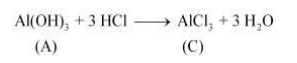Also, when compound $A$ is heated strongly, it gives compound $D$. This compound is used to extract metal X. Aluminium metal is extracted from alumina. Hence, compound D must be alumina.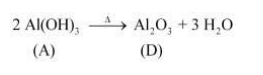Question 29: What do you understand by (a) inert pair effect (b) allotropy and (c) catenation?

Solution. (a) Inert pair effect

As one moves down the group, the tendency of $s$ -block electrons to participate in chemical bonding decreases. This effect is known as inert pair effect. In case of group 13 elements, the electronic configuration is $n s^{2} n p^{1}$ and their group valency is $+3 .$ However, on moving down the group, the $+1$ oxidation state becomes more stable. This happens because of the poor shielding of the $n s^{2}$ electrons by the $d$ - and $f$ - electrons. As a result of the poor shielding, the $n s^{2}$ electrons are held tightly by the nucleus and so, they cannot participate in chemical bonding.

(b) Allotropy

Allotropy is the existence of an element in more than one form, having the same chemical properties but different physical properties. The various forms of an element are called allotropes. For example, carbon exists in three allotropic forms: diamond, graphite, and fullerenes.

(c) Catenation

The atoms of some elements (such as carbon) can link with one another through strong covalent bonds to form long chains or branches. This property is known as catenation. It is most common in carbon and quite significant in Si and $\mathrm{S}$.

Question 30: A certain salt $X$, gives the following results.

(i) Its aqueous solution is alkaline to litmus.

(ii) It swells up to a glassy material $Y$ on strong heating.

(iii) When conc. $\mathrm{H}_{2} \mathrm{SO}_{4}$ is added to a hot solution of $X$, white crystal of an acid $Z$ separates out.

Write equations for all the above reactions and identify $X, Y$ and $Z$.

Solution. The given salt is alkaline to litmus. Therefore, $X$ is a salt of a strong base and a weak acid. Also, when $X$ is strongly heated, it swells to form substance $Y$. Therefore, $X$ must be borax. When borax is heated, it loses water and swells to form sodium metaborate. When heating is continued, it solidifies to form a glassy material $Y$. Hence, $Y$ must be a mixture of sodium metaborate and boric anhydride.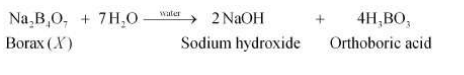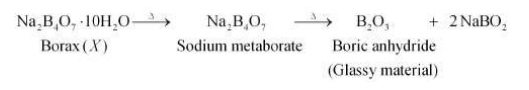When concentrated acid is added to borax, white crystals of orthoboric acid $(Z)$ are formed.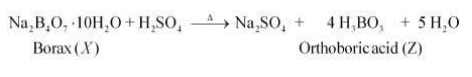Question 31: Write balanced equations for:

(i) $\mathrm{BF}_{3}+\mathrm{LiH} \rightarrow$

(ii) $\mathrm{B}_{2} \mathrm{H}_{6}+\mathrm{H}_{2} \mathrm{O} \rightarrow$

(iii) $\mathrm{NaH}+\mathrm{B}_{2} \mathrm{H}_{6} \rightarrow$

(iv) $\mathrm{H}_{3} \mathrm{BO}_{3} \stackrel{\Delta}{\longrightarrow}$

(v) $\mathrm{Al}+\mathrm{NaOH} \rightarrow$

$(\mathrm{vi}) \mathrm{B}_{2} \mathrm{H}_{6}+\mathrm{NH}_{3} \rightarrow$

Solution.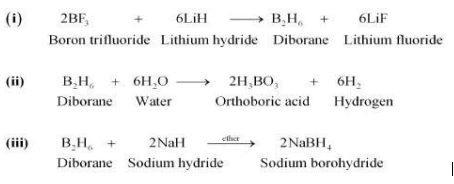(iv)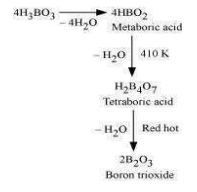(v) $2 \mathrm{Al}+2 \mathrm{NaOH}+6 \mathrm{H}_{2} \mathrm{O}$

$\longrightarrow 2 \mathrm{Na}^{+}\left[\mathrm{Al}(\mathrm{OH})_{4}\right]_{(\mathrm{oq})}^{-}+3 \mathrm{H}_{2}$

Sodium tetrahydroxoaluminate (III)

(vi) $3 \mathrm{~B}_{2} \mathrm{H}_{6}+6 \mathrm{NH}_{3} \longrightarrow 3\left[\mathrm{BH}_{2}\right.$

$\left.\left(\mathrm{NH}_{3}\right)_{2}\right]\left[\mathrm{BH}_{4}\right] \stackrel{2}{\longrightarrow} \mathrm{B}_{3} \mathrm{~N}_{3} \mathrm{H}_{6}+12 \mathrm{H}_{2}$

Borazene

Question 32: Give one method for industrial preparation and one for laboratory preparation of CO and

$\mathrm{CO}_{2}$ each.

Solution. Caron dioxide

In the laboratory, $\mathrm{CO}_{2}$ can be prepared by the action of dilute hydrochloric acid on calcium carbonate. The reaction involved is as follows:

$\mathrm{CaCO}_{3}+2 \mathrm{HCl}_{(a q)} \longrightarrow$$\mathrm{CaCl}_{2(\text { aq })}$

$+\mathrm{CO}_{2(g)}+\mathrm{H}_{2} \mathrm{O}_{(j)}$

$\mathrm{CO}_{2}$ is commercially prepared by heating limestone. The reaction involved is as follows:

$\mathrm{CaCO}_{3} \stackrel{\mathrm{A}}{\longrightarrow} \mathrm{CaO}+\mathrm{CO}_{2} \uparrow$

Caron monoxide

In the laboratory, $\mathrm{CO}$ is prepared by the dehydration of formic acid with conc. $\mathrm{H}_{2} \mathrm{SO}_{4}$, at $373 \mathrm{~K}$. The reaction involved is as follows:

$\mathrm{HCOOH} \frac{\mathrm{MmK}}{\operatorname{coc} \mathrm{H}_{2} \mathrm{SO}_{4}}{\longrightarrow} \mathrm{H}_{2} \mathrm{O}+\mathrm{CO} \uparrow$

CO is commercially prepared by passing steam over hot coke. The reaction involved is as follows: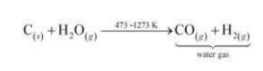Question 33: An aqueous solution of borax is

(a) neutral

(b) amphoteric

(c) basic

(d) acidic

Solution. (c) Borax is a salt of a strong base ( $\mathrm{NaOH}$ ) and a weak acid $\left(\mathrm{H}_{3} \mathrm{BO}_{3}\right) .$ It is, therefore, basic in nature.

Question 34: Boric acid is polymeric due to

(a) its acidic nature

(b) the presence of hydrogen bonds

(c) its monobasic nature

(d) its geometry

Solution. (b) Boric acid is polymeric because of the presence of hydrogen bonds. In the given

figure, the dotted lines represent hydrogen bonds.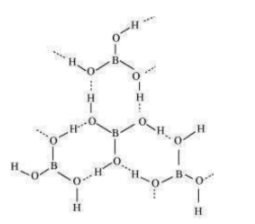Question 35: The type of hybridisation of boron in diborane is

(a) $s p$

(b) $s p^{2}$

(c) $s p^{3}$

(d) $d s p^{2}$

Solution. (c) Boron in diborane is $s p^{3}$ hybridised.

Question 36: Thermodynamically the most stable form of carbon is

(a) diamond

(b) graphite

(c) fullerenes

(d) coal

Solution. (b) Graphite is thermodynamically the most stable form of carbon.

Question 37: Elements of group 14

(a) exhibit oxidation state of $+4$ only

(b) exhibit oxidation state of $+2$ and $+4$

(c) form $\mathrm{M}^{2-}$ and $\mathrm{M}^{4+}$ ion

(d) form $\mathrm{M}^{2+}$ and $\mathrm{M}^{4+}$ ions

Solution. (b) The elements of group 14 have 4 valence electrons. Therefore, the oxidation state of the group is $+4$. However, as a result of the inert pair effect, the lower oxidation state becomes more and more stable and the higher oxidation state becomes less stable.

Therefore, this group exhibits $+4$ and $+2$ oxidation states.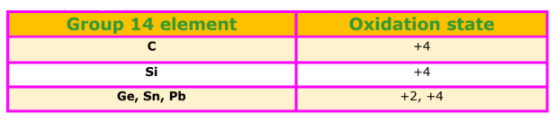Question 38: If the starting material for the manufacture of silicones is $\mathrm{RSiCl}_{3}$, write the structure of the product formed.

Solution. $\mathrm{RSiCl}_{3}+3 \mathrm{H}_{2} \mathrm{O} \longrightarrow \mathrm{RSi}(\mathrm{OH})_{3}+3 \mathrm{HCl}$

(i)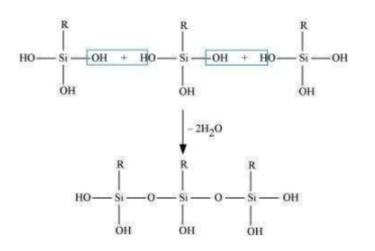(ii)